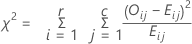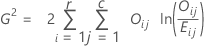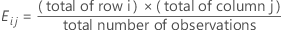# Methods and formulas for chi-square test for association for Cross Tabulation and Chi-Square

Select the method or formula of your choice.

## Chi-square test

### Formula

Minitab displays two chi-square statistics.

Pearson chi-square statistic:Likelihood-ratio chi-square statistic:###### Note

If any cell has an expected frequency less than one, the p-value for the test is not displayed because the results may not be valid. If some cells have small expected frequencies, consider combining or omitting row and/or column categories.

Yates' correction for 2 x 2 tables is not used.

### Notation

TermDescription
Oijobserved frequency in cell (i, j)
Eij

expected frequency for cell (i, j)## Expected cell count

The expected count is the frequency that would be expected in a cell, on average, if the variables are independent. Minitab calculates the expected counts as the product of the row and column totals, divided by the total number of observations.

### Notation

TermDescription
ni+ number of observations in the ith row
n+j number of observations in the jth column
n++ total number of observations

## Raw residual calculation

### Formula

Raw residual = Oij – Eij

### Notation

TermDescription
Oij observed frequency in cell (i, j)
Eij expected frequency for cell (i, j)

## Standardized residual calculation

### Notation

TermDescription
Oij observed frequency in cell (i, j)
Eij expected frequency for cell (i, j)

### Notation

TermDescription
Oij observed frequency in cell (i, j)
Eij expected frequency for cell (i, j)
ni+ number of observations in the ith row
n+j number of observations in the jth column
n++ total number of observations

## Contribution to chi-square statistic from each cell

### Formula

The contribution to the χ2 statistic from each cell is:

### Notation

TermDescription
Oij observed frequency in cell (i, j)
Eij expected frequency in cell (i, j)